# Congruent triangles class 9 worksheet with answers

Given below are the Class 9 Maths Triangle congruence worksheet with answers
(a) Concepts questions
(b) Calculation problems
(c) Multiple choice questions
(e) Fill in the blanks
(f)Subjective Questions

## Subjective Questions

Question 1
Let ABC and PQR are two triangles.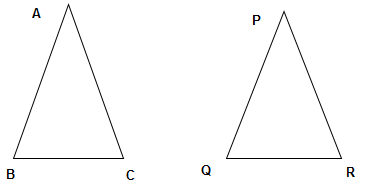Which cases the triangles will be congruent? Write down the congruence equation
1. $AB =PQ \; , \; BC=QR \; and \; AC=PR$
2. $BC=QR \; , \; \angle B= \angle Q \; , \;\angle C=\angle R$
3. $AB=PQ, BC=QR, \angle C= \angle P$
4. $AB=PQ, BC=QR, \angle B= \angle Q$
5. $AB=PQ \; , \; \angle A= \angle P \;,\; \angle C= \angle R$
6. $AB =PQ \; , \; BC=QR$
7. $\angle B= \angle Q \; , \; \angle C= \angle R$
8. $\angle A= \angle P, \angle B=\angle Q ,\angle C= \angle R$
9. $AB=PR , \angle A= \angle P, \angle B=\angle R$

## True or False statement

Question 2
True or False statement
a. We cannot construct a triangle of side 9,5,4 cm
b. In a Right angle triangle, hyponotuse is the longest side c. centroid is the point of intersection of the median of the triangles
d. A triangle can have two obtuse angles
e. Orthocenter is the point of intersection of altitudes
f. if $ABC \cong PQR$ then AC=PQ
g. if a triangle ABC such that AB > BC , Then $\angle C > \angle A$
h. Mid point of the hypotenuse of right angled triangle is circumcenter

## Multiple choice Questions

Question 4
In a triangle ABC , $\angle A=60^0$ ,#\angle B=40^0$, which side is the longest a. AB b. BC c. AC Question 5 In triangle ABC , AB=AC and D is the point inside triangle such that BD=DC as shown in figure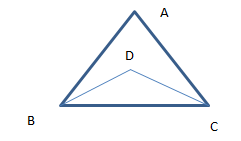Which of the following is true a.$\Delta ABD \cong \Delta ACD$b.$\angle ABD= \angle ACD$c.$\angle DAC= \angle DAB$d. All the above Question 6 An exterior angle of the triangle is$110^0$, One of the opposite interior angle is$50^0$What are the other two angles a.$60^0,70^0$b.$55^0,55^0$c.$70^0,50^0$d. None of the above Question 7 AD is the median of the triangle . Which of the following is true?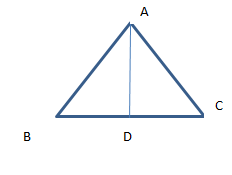a.$AB+BC+AC > 2AD$b.$AB +BC > AC$c.$AB+BC+AC> AD$d. none of these Question 8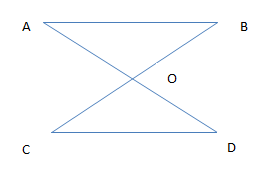In the above figure AB || CD ,O is the mid point BC. Which of the following is true a.$ \Delta AOB \cong \Delta DOC$b. O is the mid point of AD c.$AB=CD$d. All the above Question 9 In an isosceles triangle ΔABC with AB = AC. D is mid point on BC. Which of the following is true a. Orthocenter lies on line AD b. AD is the perpendicular bisector BC c. Centroid lies on the line AD d. AD is the bisector of angle A Question 10 PQR is a right angle triangle in with$P=90^0$and PQ=PR . What is the value of Q and R a.$45^0,45^0$b.$30^0,60^0$c.$20^0,60^0$Question 11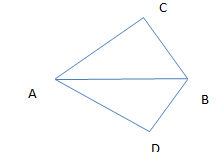In the above quadrilateral ACBD, we have AC=AD and AB bisect the ∠A Which of the following is true a.$ \Delta ABC \cong \Delta ABD$b. BC=BD c.$ \angle C= \angle D\$
d. None of the above

## Match the column

Question 12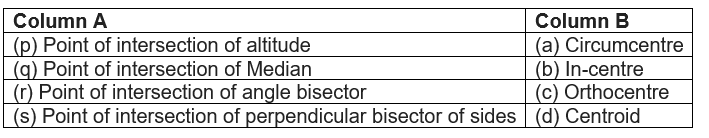Question 13
PQ > PR and QS and RS are the bisectors of angle Q and R, respectively. Show that SQ > SR.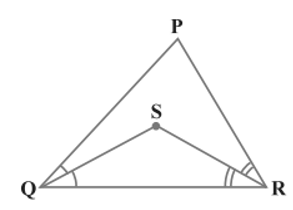Question 14
l || m and M is the mid-point of a line segment AB. Show that M is also the mid-point of any line segment CD, having its end points on l and m, respectively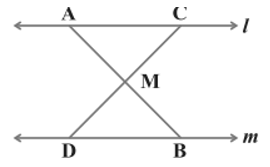Question 15
S is any point in the interior of triangle PQR. Show that SQ + SR < PQ + PR

## Summary

This Congruent triangles class 9 worksheet with answers is prepared keeping in mind the latest syllabus of CBSE . This has been designed in a way to improve the academic performance of the students. If you find mistakes , please do provide the feedback on the mail. You can download this test as pdf also as below